# Search by Topic

Filter by: Content type:
Age range:
Challenge level:

### There are 42 results

Broad Topics > 3D Geometry, Shape and Space > Polyhedra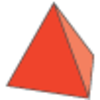### Next Size Up

##### Age 7 to 11 Challenge Level:

The challenge for you is to make a string of six (or more!) graded cubes.### Octa-flower

##### Age 16 to 18 Challenge Level:

Join some regular octahedra, face touching face and one vertex of each meeting at a point. How many octahedra can you fit around this point?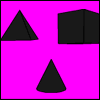##### Age 5 to 7 Challenge Level:

Here are shadows of some 3D shapes. What shapes could have made them?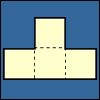### Cut Nets

##### Age 7 to 11 Challenge Level:

Each of the nets of nine solid shapes has been cut into two pieces. Can you see which pieces go together?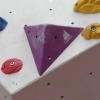### Tetra Perp

##### Age 16 to 18 Challenge Level:

Show that the edges AD and BC of a tetrahedron ABCD are mutually perpendicular when: AB²+CD² = AC²+BD².### Pythagoras for a Tetrahedron

##### Age 16 to 18 Challenge Level:

In a right-angled tetrahedron prove that the sum of the squares of the areas of the 3 faces in mutually perpendicular planes equals the square of the area of the sloping face. A generalisation. . . .### Triangles to Tetrahedra

##### Age 11 to 14 Challenge Level:

Imagine you have an unlimited number of four types of triangle. How many different tetrahedra can you make?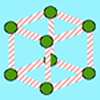### Skeleton Shapes

##### Age 5 to 7 Challenge Level:

How many balls of modelling clay and how many straws does it take to make these skeleton shapes?### Tet-trouble

##### Age 14 to 16 Challenge Level:

Is it possible to have a tetrahedron whose six edges have lengths 10, 20, 30, 40, 50 and 60 units?### Three Cubes

##### Age 14 to 16 Challenge Level:

Can you work out the dimensions of the three cubes?### More Dicey Decisions

##### Age 16 to 18 Challenge Level:

The twelve edge totals of a standard six-sided die are distributed symmetrically. Will the same symmetry emerge with a dodecahedral die?### Which Solids Can We Make?

##### Age 11 to 14 Challenge Level:

Interior angles can help us to work out which polygons will tessellate. Can we use similar ideas to predict which polygons combine to create semi-regular solids?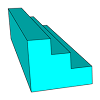### Which Solid?

##### Age 7 to 16 Challenge Level:

This task develops spatial reasoning skills. By framing and asking questions a member of the team has to find out what mathematical object they have chosen.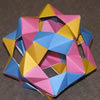### Plaited Origami Polyhedra

##### Age 7 to 16 Challenge Level:

These models have appeared around the Centre for Mathematical Sciences. Perhaps you would like to try to make some similar models of your own.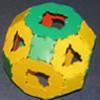### Platonic and Archimedean Solids

##### Age 7 to 16 Challenge Level:

In a recent workshop, students made these solids. Can you think of reasons why I might have grouped the solids in the way I have before taking the pictures?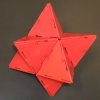### Investigating Solids with Face-transitivity

##### Age 14 to 18

In this article, we look at solids constructed using symmetries of their faces.### Lighting up Time

##### Age 7 to 14 Challenge Level:

A very mathematical light - what can you see?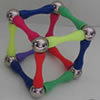### Magnetic Personality

##### Age 7 to 16 Challenge Level:

60 pieces and a challenge. What can you make and how many of the pieces can you use creating skeleton polyhedra?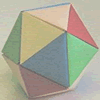### Paper Folding - Models of the Platonic Solids

##### Age 11 to 16

A description of how to make the five Platonic solids out of paper.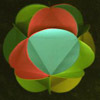### Making Maths: Triangular Ball

##### Age 7 to 11 Challenge Level:

Make a ball from triangles!### Sliced

##### Age 14 to 16 Challenge Level:

An irregular tetrahedron has two opposite sides the same length a and the line joining their midpoints is perpendicular to these two edges and is of length b. What is the volume of the tetrahedron?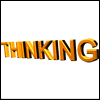### Thinking 3D

##### Age 7 to 14

How can we as teachers begin to introduce 3D ideas to young children? Where do they start? How can we lay the foundations for a later enthusiasm for working in three dimensions?### Tetrahedra Tester

##### Age 11 to 14 Challenge Level:

An irregular tetrahedron is composed of four different triangles. Can such a tetrahedron be constructed where the side lengths are 4, 5, 6, 7, 8 and 9 units of length?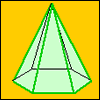### A Chain of Eight Polyhedra

##### Age 7 to 11 Challenge Level:

Can you arrange the shapes in a chain so that each one shares a face (or faces) that are the same shape as the one that follows it?### The Dodecahedron

##### Age 16 to 18 Challenge Level:

What are the shortest distances between the centres of opposite faces of a regular solid dodecahedron on the surface and through the middle of the dodecahedron?### Dodecawhat

##### Age 14 to 16 Challenge Level:

Follow instructions to fold sheets of A4 paper into pentagons and assemble them to form a dodecahedron. Calculate the error in the angle of the not perfectly regular pentagons you make.### Euler's Formula and Topology

##### Age 16 to 18

Here is a proof of Euler's formula in the plane and on a sphere together with projects to explore cases of the formula for a polygon with holes, for the torus and other solids with holes and the. . . .### Classifying Solids Using Angle Deficiency

##### Age 11 to 16 Challenge Level:

Toni Beardon has chosen this article introducing a rich area for practical exploration and discovery in 3D geometry### The Dodecahedron Explained

##### Age 16 to 18

What is the shortest distance through the middle of a dodecahedron between the centres of two opposite faces?### Icosian Game

##### Age 11 to 14 Challenge Level:

This problem is about investigating whether it is possible to start at one vertex of a platonic solid and visit every other vertex once only returning to the vertex you started at.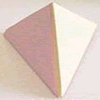### Child's Play

##### Age 7 to 11 Challenge Level:

A toy has a regular tetrahedron, a cube and a base with triangular and square hollows. If you fit a shape into the correct hollow a bell rings. How many times does the bell ring in a complete game?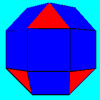### Rhombicubocts

##### Age 11 to 14 Challenge Level:

Each of these solids is made up with 3 squares and a triangle around each vertex. Each has a total of 18 square faces and 8 faces that are equilateral triangles. How many faces, edges and vertices. . . .### Tetra Square

##### Age 11 to 14 Challenge Level:

ABCD is a regular tetrahedron and the points P, Q, R and S are the midpoints of the edges AB, BD, CD and CA. Prove that PQRS is a square.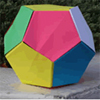### Dodecamagic

##### Age 7 to 11 Challenge Level:

Here you see the front and back views of a dodecahedron. Each vertex has been numbered so that the numbers around each pentagonal face add up to 65. Can you find all the missing numbers?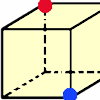### Redblue

##### Age 7 to 11 Challenge Level:

Investigate the number of paths you can take from one vertex to another in these 3D shapes. Is it possible to take an odd number and an even number of paths to the same vertex?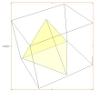### A Mean Tetrahedron

##### Age 11 to 14 Challenge Level:

Can you number the vertices, edges and faces of a tetrahedron so that the number on each edge is the mean of the numbers on the adjacent vertices and the mean of the numbers on the adjacent faces?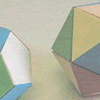### Face Painting

##### Age 7 to 11 Challenge Level:

You want to make each of the 5 Platonic solids and colour the faces so that, in every case, no two faces which meet along an edge have the same colour.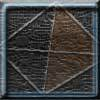### Tetrahedron Faces

##### Age 7 to 11 Challenge Level:

One face of a regular tetrahedron is painted blue and each of the remaining faces are painted using one of the colours red, green or yellow. How many different possibilities are there?### Tetra Inequalities

##### Age 16 to 18 Challenge Level:

Prove that in every tetrahedron there is a vertex such that the three edges meeting there have lengths which could be the sides of a triangle.### Reach for Polydron

##### Age 16 to 18 Challenge Level:

A tetrahedron has two identical equilateral triangles faces, of side length 1 unit. The other two faces are right angled isosceles triangles. Find the exact volume of the tetrahedron.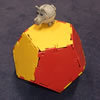### Platonic Planet

##### Age 14 to 16 Challenge Level:

Glarsynost lives on a planet whose shape is that of a perfect regular dodecahedron. Can you describe the shortest journey she can make to ensure that she will see every part of the planet?### Proximity

##### Age 14 to 16 Challenge Level:

We are given a regular icosahedron having three red vertices. Show that it has a vertex that has at least two red neighbours.